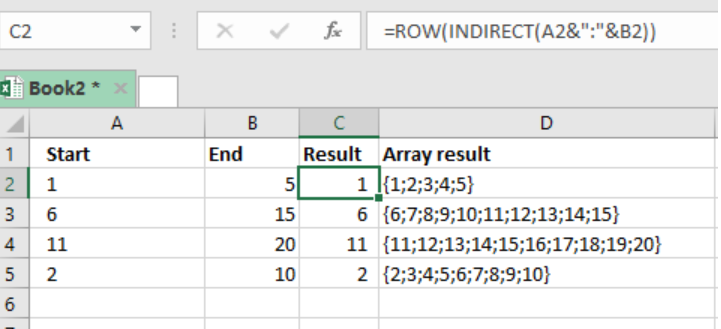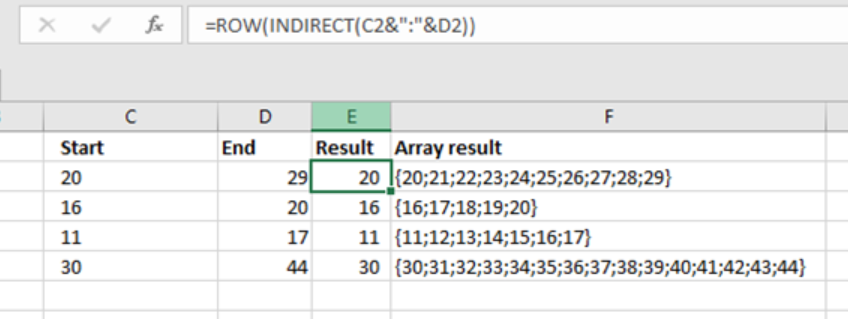Get instant live expert help with Excel or Google Sheets“My Excelchat expert helped me in less than 20 minutes, saving me what would have been 5 hours of work!”

#### Post your problem and you'll get expert help in seconds

Your message must be at least 40 characters
Our professional experts are available now. Your privacy is guaranteed.

# Create Array of Numbers

We can use the ROW and INDIRECT functions to create an array of numbers, e.g. {1; 2; 3; 4}. The functions are mostly used in array formulas requiring a numeric array for processing. This post provides a guide on how to create array of numbers using the ROW and INDIRECT functions.Figure 1: How to create array of numbers in excel

## Syntax of the formula

`{=ROW (INDIRECT (start&”:”&end))}`

## How the formula works

• ROW and INDIRECT functions are fundamental when we want to create an array of numbers
• We need to state the start and end of the array as shown in the example above
• Then in the result column, in put the formula; `=ROW(INDIRECT(start&”:”&end)))`
• Then press “Enter”

Note that if the column length is not long enough, we only get the start value in the array. To get the array, we create another column; “Array result”. Then, take the formula to formula bar, and click the F9 function to get the array results.

## Understanding the formula

A string that represents the rows forms the core of this formula. For us to create an array with 5 numbers, we need to hard-core a string into the INDIRECT function. This can be done as below:

`=ROW(INDIRECT(“1:5”))`

Here, the formula works as:

• INDIRECT interprets it to mean range 1:5 (5 rows)
• ROW function returns row number for each row inside the array

## ExampleFigure 2: Creating an array of numbers in excel

In the example above, we proceed as follows:

• Specify the start and end data in the sheet. Also indicate where the result should be as well as the array result.
• In the first row where we have the data, below in the result column, specify the formula, i.e. =ROW(INDIRECT(C2&”:”&D2))
• Press Enter to get the result for the first row.
• Use the F9 key to get the array of the data in the “array result ” column

## Instant Connection to an Expert through our Excelchat Service

Most of the time, the problem you will need to solve will be more complex than a simple application of a formula or function. If you want to save hours of research and frustration, try our live Excelchat service! Our Excel Experts are available 24/7 to answer any Excel question you may have. We guarantee a connection within 30 seconds and a customized solution within 20 minutes.

### Did this post not answer your question? Get a solution from connecting with the expert.Another blog reader asked this question today on Excelchat:## Subscribe to Excelchat.coAnother blog reader asked this question today on Excelchat: